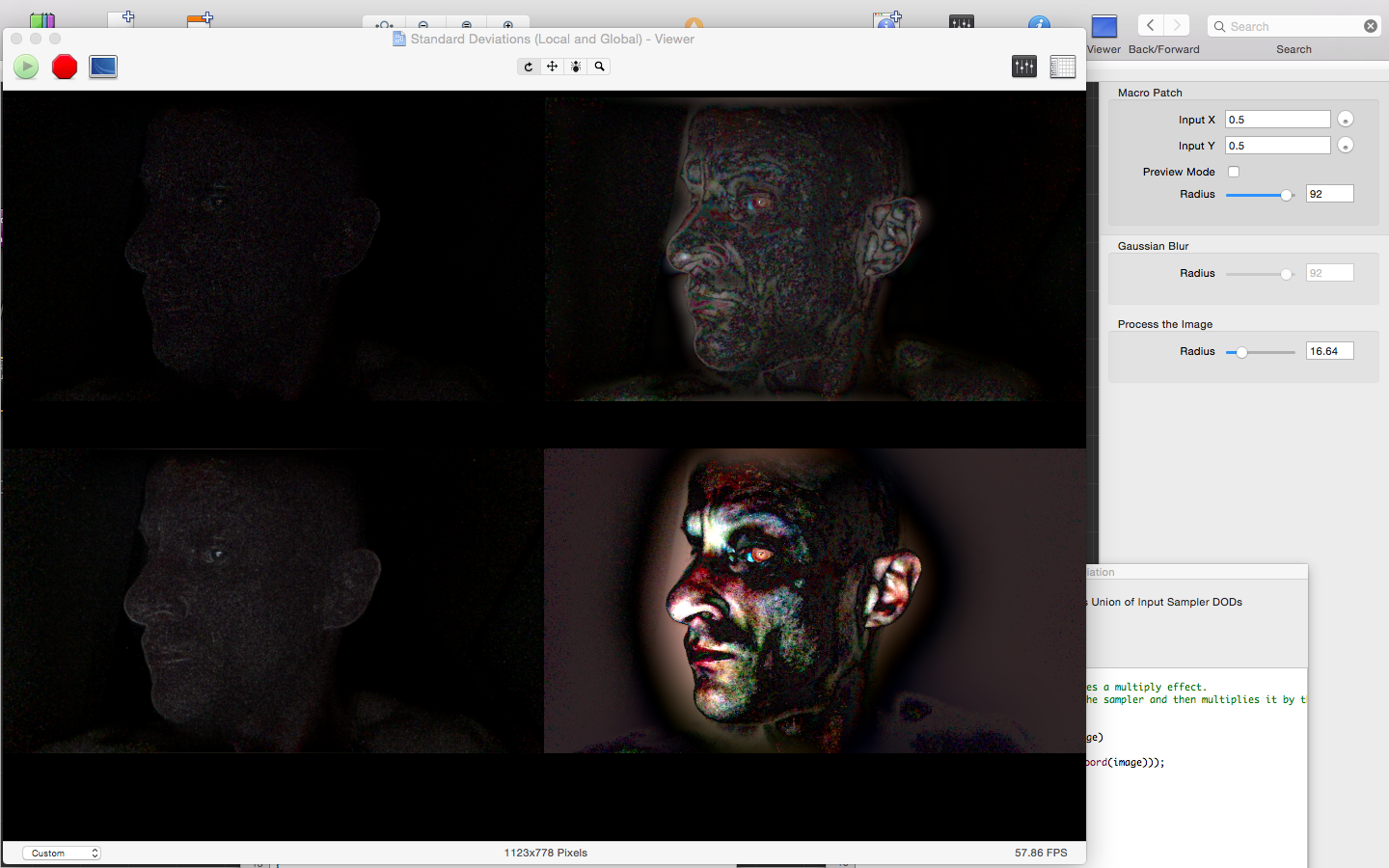## Sunday, May 3, 2015

### TECHNOLOGY | Isolating chroma using variance and standard deviation statistics

Coming up
By calculating the distribution of pixels and their variance from the mean (or average), and then displaying only pixels with a wide variance, unusual elements in an image, e.g., demonic entities and related activity, can be isolated or enhanced:From left, clockwise: Median-calculated standard deviation, adjustable Gaussian blur-standard deviation, 1 x 1 nearest neighbor-averaged standard deviation, global (mean) standard deviation
Check back soon for code that calculates the variance and standard deviation statistics for an image in OpenGL ES 3.0 and OpenCV:The code and image-processing pipeline for the Gaussian-blur (local adaptive) standard deviation calculation
The post will also present methods for further refinement of images generated by variance and standard deviation statistics:Other image-processing procedures can be applied to enhance the results of images created using variance/standard deviation statistics